# 深度学习中的Attention机制

【GiantPandaCV导语】 近几年，Attention-based方法因其可解释和有效性，受到了学术界和工业界的欢迎。但是，由于论文中提出的网络结构通常被嵌入到分类、检测、分割等代码框架中，导致代码比较冗余，对于像我这样的小白很难找到网络的核心代码，导致在论文和网络思想的理解上会有一定困难。因此，我把最近看的Attention、MLP和Re-parameter论文的核心代码进行了整理和复现，方便各位读者理解。本文主要对该项目的Attention部分做简要介绍。项目会持续更新最新的论文工作，欢迎大家follow和star该工作，若项目在复现和整理过程中有任何问题，欢迎大家在issue中提出，我会及时回复~

## 项目地址¶

https://github.com/xmu-xiaoma666/External-Attention-pytorch

## 1. External Attention¶

### 1.1. 引用¶

Beyond Self-attention: External Attention using Two Linear Layers for Visual Tasks.---arXiv 2021.05.05

### 1.2. 模型结构¶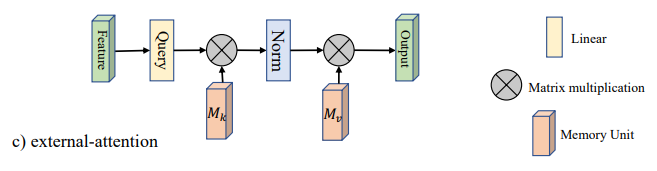### 1.4. 使用方法¶

from attention.ExternalAttention import ExternalAttention
import torch

input=torch.randn(50,49,512)
ea = ExternalAttention(d_model=512,S=8)
output=ea(input)
print(output.shape)


## 2. Self Attention¶

### 2.1. 引用¶

Attention Is All You Need---NeurIPS2017

### 2.2. 模型结构¶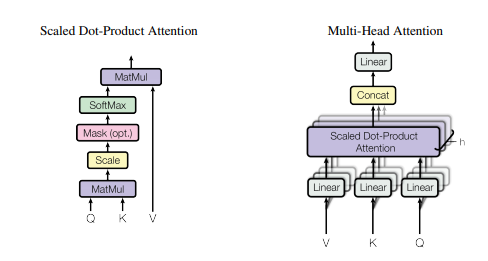### 2.4. 使用方法¶

from attention.SelfAttention import ScaledDotProductAttention
import torch

input=torch.randn(50,49,512)
sa = ScaledDotProductAttention(d_model=512, d_k=512, d_v=512, h=8)
output=sa(input,input,input)
print(output.shape)


## 3. Squeeze-and-Excitation(SE) Attention¶

### 3.1. 引用¶

Squeeze-and-Excitation Networks---CVPR2018

### 3.2. 模型结构¶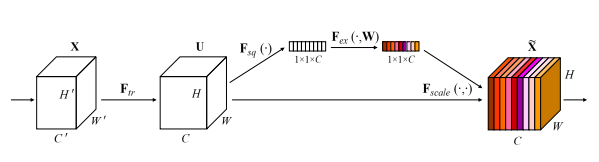### 3.4. 使用方法¶

from attention.SEAttention import SEAttention
import torch

input=torch.randn(50,512,7,7)
se = SEAttention(channel=512,reduction=8)
output=se(input)
print(output.shape)


## 4. Selective Kernel(SK) Attention¶

### 4.1. 引用¶

Selective Kernel Networks---CVPR2019

### 4.2. 模型结构¶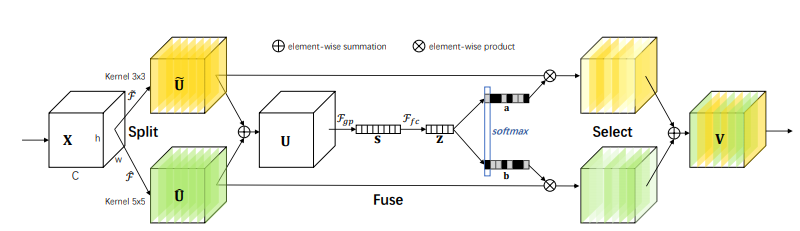### 4.4. 使用方法¶

from attention.SKAttention import SKAttention
import torch

input=torch.randn(50,512,7,7)
se = SKAttention(channel=512,reduction=8)
output=se(input)
print(output.shape)


## 5. CBAM Attention¶

### 5.1. 引用¶

CBAM: Convolutional Block Attention Module---ECCV2018

### 5.2. 模型结构¶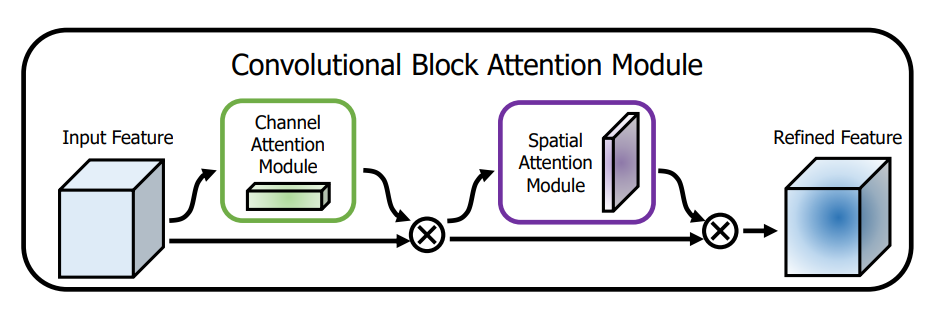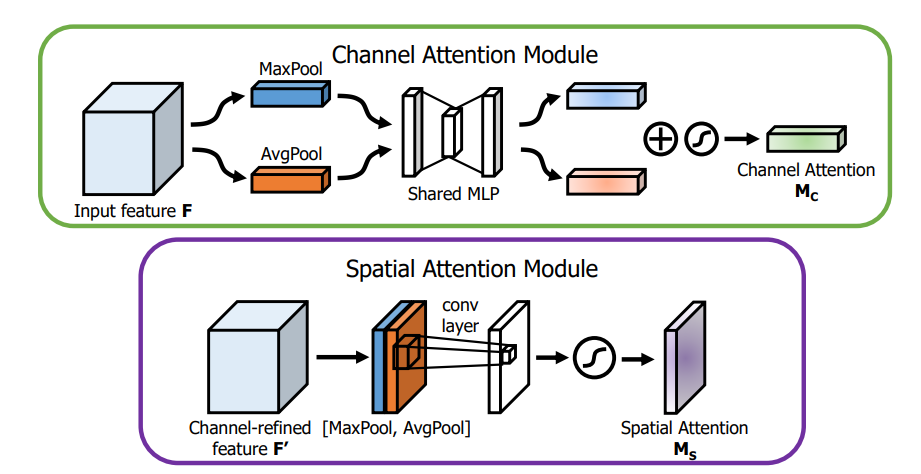### 5.3. 简介¶

Channel Attention方面，大致结构还是和SE相似，不过作者提出AvgPool和MaxPool有不同的表示效果，所以作者对原来的特征在Spatial维度分别进行了AvgPool和MaxPool，然后用SE的结构提取channel attention，注意这里是参数共享的，然后将两个特征相加后做归一化，就得到了注意力矩阵。

Spatial Attention和Channel Attention类似，先在channel维度进行两种pool后，将两个特征进行拼接，然后用7x7的卷积来提取Spatial Attention（之所以用7x7是因为提取的是空间注意力，所以用的卷积核必须足够大）。然后做一次归一化，就得到了空间的注意力矩阵。

### 5.4. 使用方法¶

from attention.CBAM import CBAMBlock
import torch

input=torch.randn(50,512,7,7)
kernel_size=input.shape
cbam = CBAMBlock(channel=512,reduction=16,kernel_size=kernel_size)
output=cbam(input)
print(output.shape)


## 6. BAM Attention¶

### 6.1. 引用¶

BAM: Bottleneck Attention Module---BMCV2018

### 6.2. 模型结构¶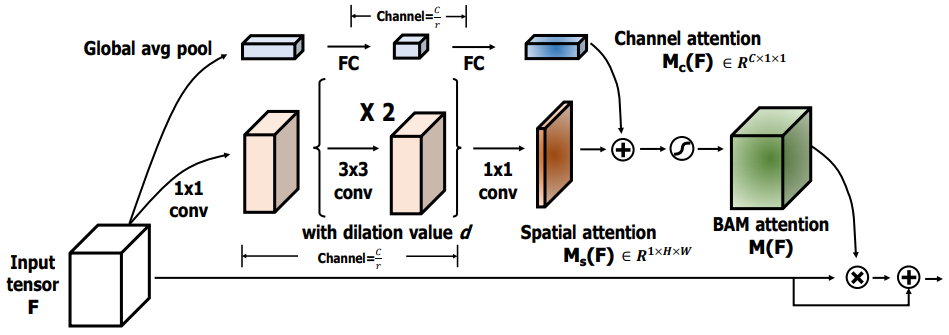### 6.3. 简介¶

Channel Attention方面，与SE的结构基本一样。Spatial Attention方面，还是在通道维度进行pool，然后用了两次3x3的空洞卷积，最后将用一次1x1的卷积得到Spatial Attention的矩阵。

### 6.4.使用方法¶

from attention.BAM import BAMBlock
import torch

input=torch.randn(50,512,7,7)
bam = BAMBlock(channel=512,reduction=16,dia_val=2)
output=bam(input)
print(output.shape)


## 7. ECA Attention¶

### 7.1. 引用¶

ECA-Net: Efficient Channel Attention for Deep Convolutional Neural Networks---CVPR2020

### 7.2. 模型结构¶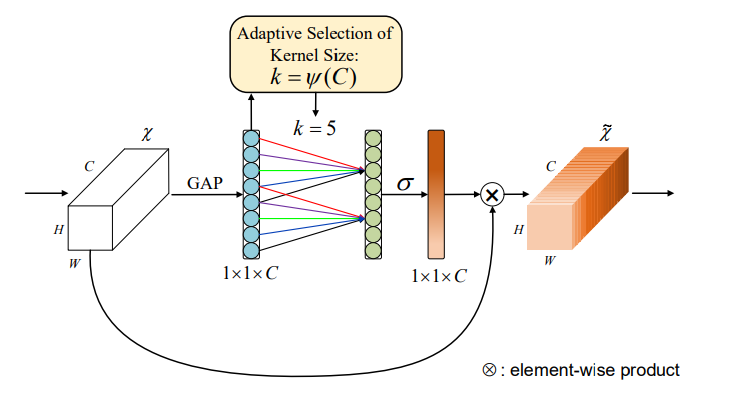### 7.4. 使用方法：¶

from attention.ECAAttention import ECAAttention
import torch

input=torch.randn(50,512,7,7)
eca = ECAAttention(kernel_size=3)
output=eca(input)
print(output.shape)


## 8. DANet Attention¶

### 8.1. 引用¶

Dual Attention Network for Scene Segmentation---CVPR2019

### 8.2. 模型结构¶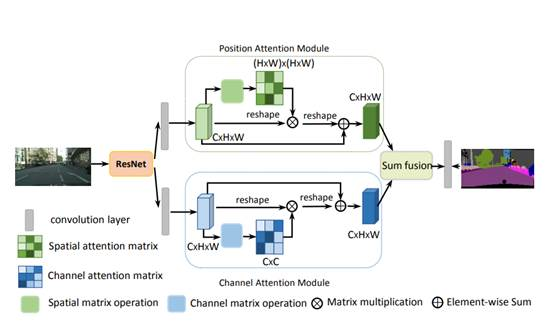### 8.4. 使用方法¶

from attention.DANet import DAModule
import torch

input=torch.randn(50,512,7,7)
danet=DAModule(d_model=512,kernel_size=3,H=7,W=7)
print(danet(input).shape)


## 9. Pyramid Split Attention(PSA)¶

### 9.1. 引用¶

EPSANet: An Efficient Pyramid Split Attention Block on Convolutional Neural Network---arXiv 2021.05.30

### 9.2. 模型结构¶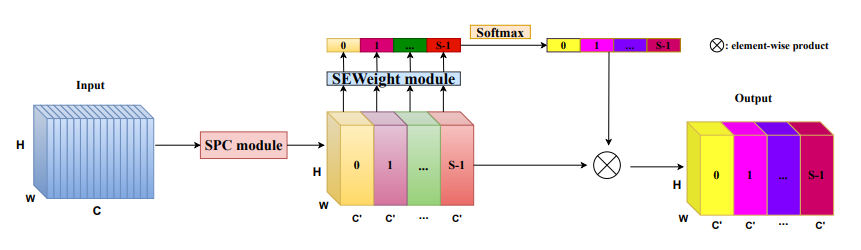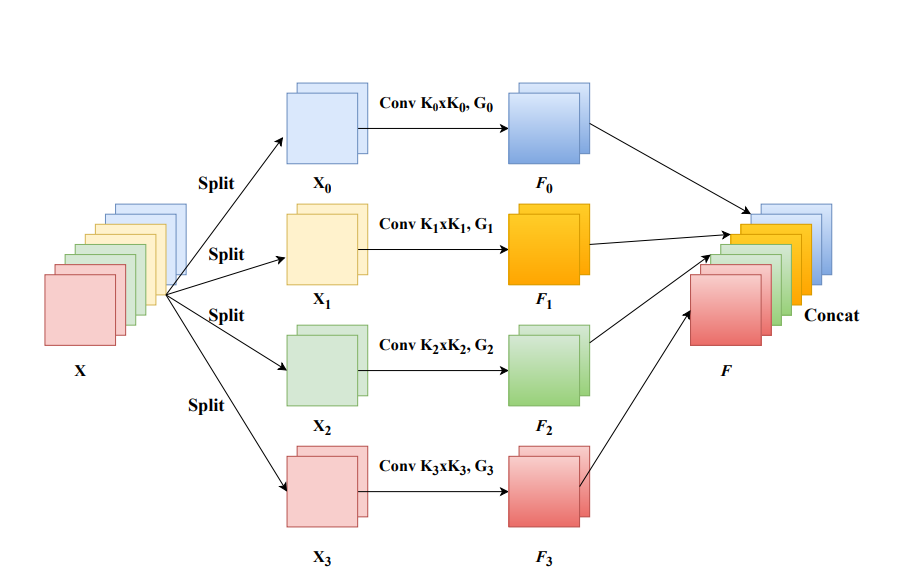### 9.4. 使用方法¶

from attention.PSA import PSAimport torchinput=torch.randn(50,512,7,7)psa = PSA(channel=512,reduction=8)output=psa(input)print(output.shape)


### 10.1. 引用¶

ResT: An Efficient Transformer for Visual Recognition---arXiv 2021.05.28

### 10.2. 模型结构¶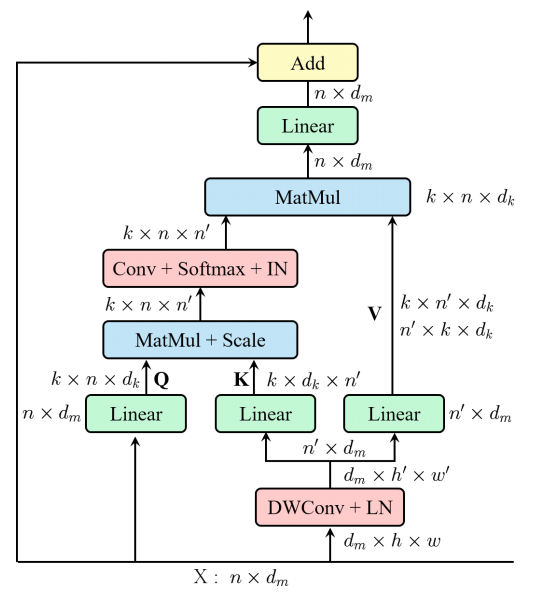### 10.4. 使用方法¶

from attention.EMSA import EMSAimport torchfrom torch import nnfrom torch.nn import functional as Finput=torch.randn(50,64,512)emsa = EMSA(d_model=512, d_k=512, d_v=512, h=8,H=8,W=8,ratio=2,apply_transform=True)output=emsa(input,input,input)print(output.shape)